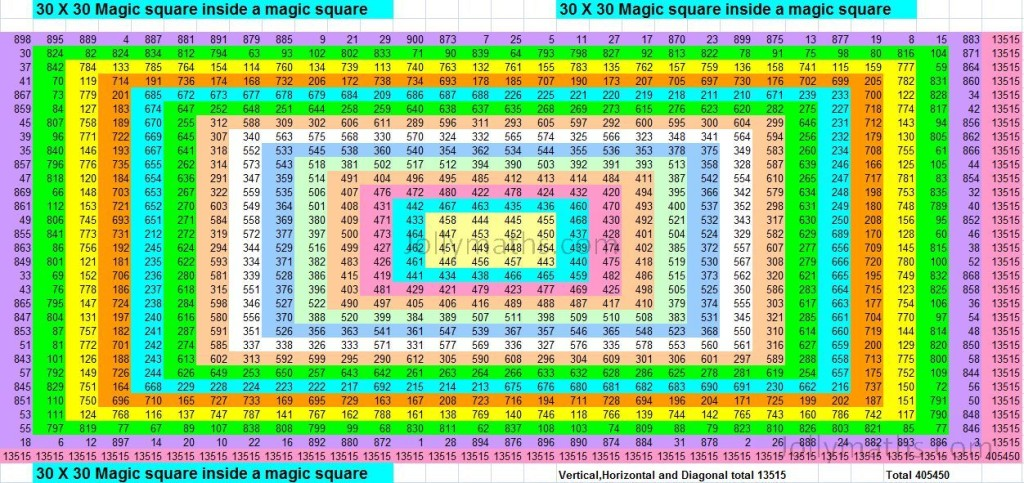# 9×54 Ramanujan sudoku

This is a variation of the popular puzzle Sudoku.

The word “RAMANUJAN” occupied 89 cells and numbers 1 to 9 are used 9 times each and the balance filled with 1 to 8. 8 along with the properties of NORMAL SUDOKU.

1) The entire word “RAMANUJAN” is first written using 119 squares (or cells).
2) Numbers 1 to 9 are filled inside each of these letters.
3) In total, the numbers 1 to 9 are written 13 times and the remaining cells are filled with 1 and 2.
4) We then take each Sudoku of 9 x 9 individually and fill them. The total number of squares that are covered for the word/letter are filled 1 to 9, the adjacent Sudoku (in the right) will commence with the numbers next to the last with 1 to 9 till it fills in the alphabet in that 9 x 9 Sudoku, and the next word/letter will start with the filled number.
For example, the first sudoku contains 20 squares, that are filled with 1 to 9 two times and the balance with 1 & 2. Hence the next Sudoku will commence with 3 to 9, 1 to 9, and so on.

# DIAMOND inlaid Magic Square

This is a DIAMOND Magic-Square wherein all the ODD-numbers are inside the Diamond shape. (Magic-Total is 65)

[table id=diamond_inlaid_5x5 /]

# 30×30 Magic Square inside a Magic Square30×30 Magic Squares inside a Magic Square

This is a special construction of a Magic Square wherein we start with a small Magic Square (centermost one in the figure) and add rows and columns around it, ensuring that we have a Magic Square at each stage. This Magic Square has been color-coded to make the Magic Squares clearer.

# SWASTIKA Magic Square

This is a special Magic Square called as the SWASTIKA Magic Square. All odd numbers are inside the SWASTIKA SYMBOL. (The Magic-Total is 65)

[table id=swastika-5×5 /]

You can find more special Magic-Squares here